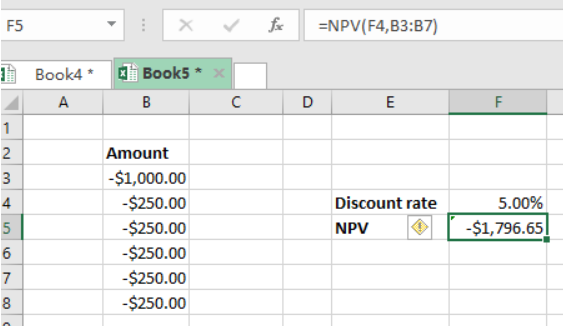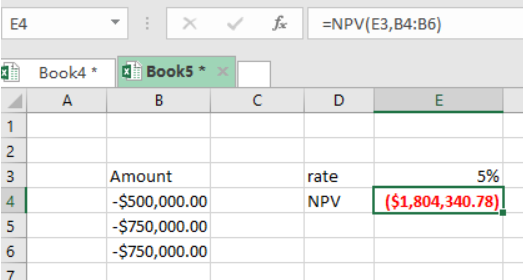Get instant live expert help with Excel or Google Sheets“My Excelchat expert helped me in less than 20 minutes, saving me what would have been 5 hours of work!”

#### Post your problem and you’ll get expert help in seconds.

Your message must be at least 40 characters
Our professional experts are available now. Your privacy is guaranteed.

# Calculating NPV in Excel with Negative Cash Flows

While calculating the net present values, a time comes when we are faced with negative cash flows. In excel, we can still get the NPV even when we have the negative cash flows. In this article, we shall learn how to calculate NPV in excel with negative cash flowsFigure 1: Calculating NPV with negative cash flows

## General syntax of the formula

`=NPV(rate, -cash flows)`

## Understanding the formula

• In excel, it is possible to get the net present value even when you only have negative cash flows.
• All you need to do is to subtract all numbers, instead of adding them.
• When you are looking to get the NPV with negative cash flows, you should also expect the NPV to be negative.
• Consider an example where the initial cost outlay is \$500,000, required rate of interest is 5% and needs additional outlays of \$750, 000 in year one or two. Here, the NPV will be negative \$500, 000 minus \$680, 272, minus 714, 285. The NPV will thus be \$1, 894, 557
• This can be done in excel as shown in the example belowFigure 2: Example of how to calculate NPV with negative cash flows

## Instant Connection to an Expert through our Excelchat Service

Most of the time, the problem you will need to solve will be more complex than a simple application of a formula or function. If you want to save hours of research and frustration, try our live Excelchat service! Our Excel Experts are available 24/7 to answer any Excel question you may have. We guarantee a connection within 30 seconds and a customized solution within 20 minutes.

Solution examplesI have a figure which is the sum of 9 months of payments. Each payment decreases by the same amount (X) so the first payment is Y, second payment is Y-X, third is Y-(Xx2), forth is Y-(Xx3) and so on. I am trying to calculate what each of the 9 payments should be.
Solved by A. W. in 60 mins